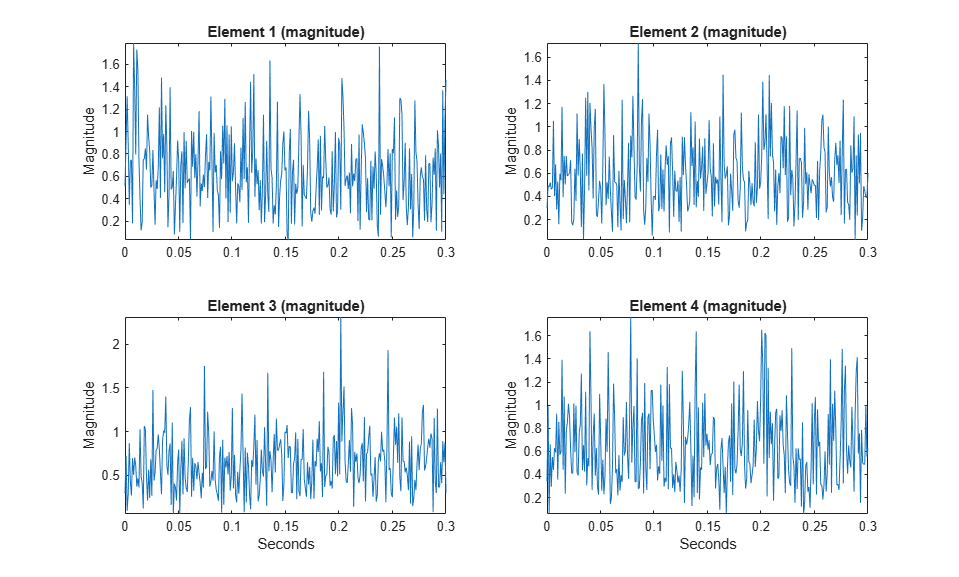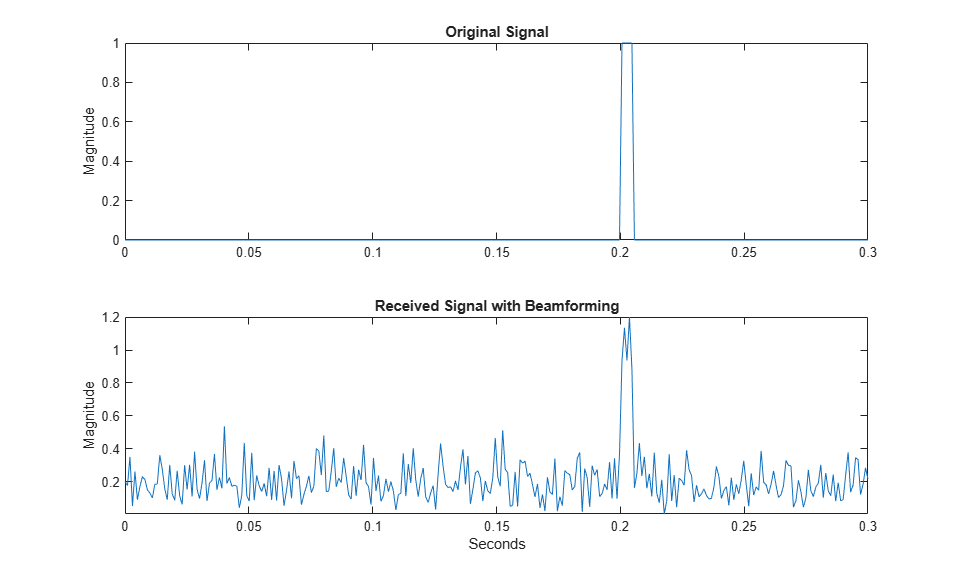## Conventional Beamforming

### Uses for Beamformers

You can use a beamformer to spatially filter the arriving signals. Accentuating or attenuating signals that arrive from specific directions helps you distinguish between signals of interest and interfering signals from other directions.

### Support for Conventional Beamforming

You can implement a narrowband phase shift beamformer using `phased.PhaseShiftBeamformer`. When you use this object, you must specify these aspects of the situation you are simulating:

• Sensor array

• Signal propagation speed

• System operating frequency

• Beamforming direction

For wideband beamformers, see Wideband Beamforming.

### Narrowband Phase Shift Beamformer For a ULA

This example shows how to create and beamform a 10-element ULA. Assume the carrier frequency is 1 GHz. Set the array element spacing to be one-half the carrier wavelength.

Note: This example runs only in R2016b or later. If you are using an earlier release, replace each call to the function with the equivalent `step` syntax. For example, replace `myObject(x)` with `step(myObject,x)`.

```fc = 1e9; lambda = physconst('LightSpeed')/fc; array = phased.ULA('NumElements',10,'ElementSpacing',lambda/2); ```

By default, the ULA elements are isotropic antennas created by the `phased.IsotropicAntennaElement` System object™. Set the frequency range of the antenna elements so that the carrier frequency lies within the operating range.

```array.Element.FrequencyRange = [8e8 1.2e9]; ```

Simulate a test signal. For this example, use a simple rectangular pulse.

```t = linspace(0,0.3,300)'; testsig = zeros(size(t)); testsig(201:205) = 1; ```

Assume the rectangular pulse is incident on the ULA from an angle of 30° azimuth and 0° elevation. Use the `collectPlaneWave` function of the ULA System object to simulate reception of the pulse waveform from the specified angle.

```angle_of_arrival = [30;0]; x = collectPlaneWave(array,testsig,angle_of_arrival,fc); ```

The signal `x` is a matrix with ten columns. Each column represents the received signal at one of the array elements.

Add complex-valued Gaussian noise to the signal `x`. Reset the default random number stream for reproducible results. Plot the magnitudes of the received pulses at the first four elements of the ULA.

```rng default npower = 0.5; x = x + sqrt(npower/2)*(randn(size(x)) + 1i*randn(size(x))); subplot(221) plot(t,abs(x(:,1))) title('Element 1 (magnitude)') axis tight ylabel('Magnitude') subplot(222) plot(t,abs(x(:,2))) title('Element 2 (magnitude)') axis tight ylabel('Magnitude') subplot(223) plot(t,abs(x(:,3))) title('Element 3 (magnitude)') axis tight xlabel('Seconds') ylabel('Magnitude') subplot(224) plot(t,abs(x(:,4))) title('Element 4 (magnitude)') axis tight xlabel('Seconds') ylabel('Magnitude') ```Construct a phase-shift beamformer. Set the `WeightsOutputPort` property to `true` to output the spatial filter weights that point the beamformer to the angle of arrival.

```beamformer = phased.PhaseShiftBeamformer('SensorArray',array,... 'OperatingFrequency',1e9,'Direction',angle_of_arrival,... 'WeightsOutputPort',true); ```

Execute the phase shift beamformer to compute the beamformer output and to compute the applied weights.

```[y,w] = beamformer(x); ```

Plot the magnitude of the output waveform along with the noise-free original waveform for comparison.

```subplot(211) plot(t,abs(testsig)) axis tight title('Original Signal') ylabel('Magnitude') subplot(212) plot(t,abs(y)) axis tight title('Received Signal with Beamforming') ylabel('Magnitude') xlabel('Seconds') ```To examine the effect of beamforming weights on the array response, plot the array normalized power response with and without beamforming weights.

```azang = -180:30:180; subplot(211) pattern(array,fc,[-180:180],0,'CoordinateSystem','rectangular',... 'Type','powerdb','PropagationSpeed',physconst('LightSpeed')) set(gca,'xtick',azang); title('Array Response without Beamforming Weights') subplot(212) pattern(array,fc,[-180:180],0,'CoordinateSystem','rectangular',... 'Type','powerdb','PropagationSpeed',physconst('LightSpeed'),... 'Weights',w) set(gca,'xtick',azang); title('Array Response with Beamforming Weights') ```